# Texas Go Math Grade 4 Lesson 4.1 Answer Key Compare Fractions Using Benchmarks

Refer to our Texas Go Math Grade 4 Answer Key Pdf to score good marks in the exams. Test yourself by practicing the problems from Texas Go Math Grade 4 Lesson 4.1 Answer Key Compare Fractions Using Benchmarks.

## Texas Go Math Grade 4 Lesson 4.1 Answer Key Compare Fractions Using Benchmarks

Essential Question

How can you use benchmarks to compare fractions?
A benchmark is a reference number that can be used to compare two fractions. If one fraction is less than the benchmark and a second fraction is greater, the first fraction is less than the second.

Unlock the Problem

Zach made a popcorn snack. He mixed $$\frac{5}{8}$$ gallon of popcorn with $$\frac{1}{2}$$ gallon of dried apple rings. Did he use more dried apple rings or more popcorn?
Zach used more dried apple rings.

Activity Compare $$\frac{5}{8}$$ and $$\frac{1}{2}$$.

Materials: fraction strips

Use traction strips to compare $$\frac{5}{8}$$ and $$\frac{1}{2}$$. Record on the model below.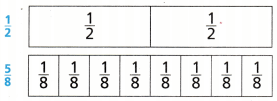$$\frac{5}{8}$$ ___________ $$\frac{1}{2}$$
So, Zach used more ______________ .
$$\frac{5}{8}$$  greater than $$\frac{1}{2}$$
So, Zach used more pop corn.
Explanation: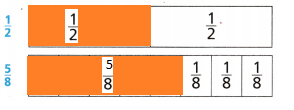Math Talk

Mathematical Processes
Explain how the number of eighth-size parts in $$\frac{5}{8}$$ is related to the number of eighth-size parts you need to make $$\frac{1}{2}$$.Explanation:
$$\frac{4}{8}$$ = $$\frac{1}{2}$$
4 eighth size parts is equal to $$\frac{1}{2}$$

Benchmarks A benchmark is a known size or amount that helps you understand a different size or amount. You can use $$\frac{1}{2}$$ as a benchmark to help you compare fractions.

Question 1.
How many eighths are equivalent to $$\frac{1}{2}$$?
4 eighth size parts is equal to $$\frac{1}{2}$$Question 2.
How can you compare $$\frac{5}{8}$$ and $$\frac{1}{2}$$ without using a model?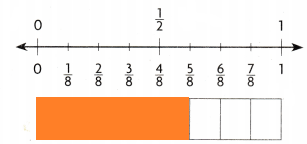By using the bench mark.
Explanation:
By using the bench marks and compare the lengths with $$\frac{1}{2}$$.
The shaded part is more than $$\frac{1}{2}$$.

Example Use benchmarks to compare fractions.

A family hiked the same mountain trail. Evie and her father hiked $$\frac{5}{12}$$ of the trail before they stopped for lunch. Jill and her mother hiked $$\frac{9}{10}$$ of the trail before they stopped for lunch. Who hiked farther before lunch?

Compare $$\frac{5}{12}$$ and $$\frac{9}{10}$$ to the benchmark $$\frac{1}{2}$$.

Step 1 Compare $$\frac{5}{12}$$ to $$\frac{1}{2}$$.Think: Shade $$\frac{5}{12}$$. $$\frac{5}{12}$$ ___________ $$\frac{1}{2}$$.Explanation:
Shaded $$\frac{5}{12}$$. $$\frac{5}{12}$$ lesser than $$\frac{1}{2}$$.

Step 2 Compare $$\frac{9}{10}$$ to $$\frac{1}{2}$$.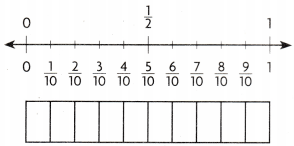Think: Shade $$\frac{9}{10}$$. $$\frac{9}{10}$$ ___________ $$\frac{1}{2}$$.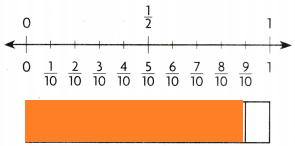Explanation:
Shaded $$\frac{9}{10}$$. $$\frac{9}{10}$$ is greater than $$\frac{1}{2}$$.

Since $$\frac{5}{12}$$ is _____________ than $$\frac{1}{2}$$ and is $$\frac{9}{10}$$ than $$\frac{1}{2}$$, you know that $$\frac{5}{12}$$ ___________ $$\frac{9}{10}$$.
So, _____________ hiked farther before lunch.
Since $$\frac{5}{12}$$ is lesser than $$\frac{1}{2}$$ and $$\frac{9}{10}$$ is greater than $$\frac{1}{2}$$, you know that $$\frac{5}{12}$$ lesser than $$\frac{9}{10}$$.
So, Jill and her mother hiked farther before lunch.

Share and show

Question 1.
Compare $$\frac{2}{5}$$ and $$\frac{1}{8}$$. Write < or >.$$\frac{2}{5}$$ ______________ $$\frac{1}{8}$$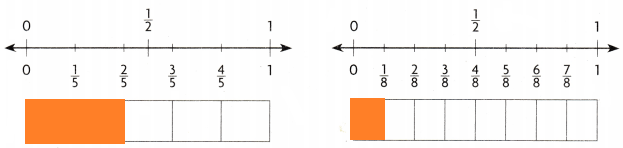Explanation:
$$\frac{2}{5}$$ greater than $$\frac{1}{8}$$
Shaded part represent the area covered

Compare. Write < or >.

Question 2.
$$\frac{1}{2}$$ ______________ $$\frac{4}{6}$$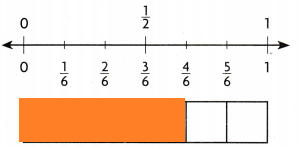Explanation:
$$\frac{1}{2}$$  is greater than $$\frac{4}{6}$$
The shaded part is more than $$\frac{1}{2}$$

Question 3.
$$\frac{3}{10}$$ ______________ $$\frac{1}{2}$$Explanation:
$$\frac{3}{10}$$ is lesser than  $$\frac{1}{2}$$
The shaded part is less than $$\frac{1}{2}$$

Question 4.
$$\frac{1}{2}$$ ______________ $$\frac{4}{8}$$Explanation:
$$\frac{1}{2}$$ is equal to $$\frac{4}{8}$$

Question 5.
$$\frac{5}{8}$$ ______________ $$\frac{2}{5}$$Explanation:
$$\frac{5}{8}$$ is greater than $$\frac{2}{5}$$

H.O.T. Algebra Find a numerator that makes the statement true.

Question 6.
$$\frac{2}{4}$$ < $$\frac{}{6}$$
$$\frac{2}{4}$$ < $$\frac{5}{6}$$
Explanation:
$$\frac{2}{4}$$ is equal to $$\frac{1}{2}$$ and $$\frac{5}{6}$$ is greater than $$\frac{1}{2}$$

Question 7.
$$\frac{8}{10}$$ < $$\frac{}{8}$$
$$\frac{8}{10}$$ < $$\frac{8}{8}$$
Explanation:
$$\frac{8}{10}$$ is greater than $$\frac{1}{2}$$ and $$\frac{8}{8}$$ is equal to 1 so greater than $$\frac{8}{10}$$

Question 8.
$$\frac{10}{12}$$ < $$\frac{}{4}$$
$$\frac{10}{12}$$ < $$\frac{4}{4}$$
Explanation:
$$\frac{10}{12}$$ is greater than $$\frac{1}{2}$$ and $$\frac{4}{4}$$ is equal to 1 so greater than $$\frac{10}{12}$$

Question 9.
$$\frac{2}{5}$$ < $$\frac{}{10}$$
$$\frac{2}{5}$$ < $$\frac{6}{10}$$
Explanation:
$$\frac{8}{10}$$ is greater than $$\frac{1}{2}$$ and $$\frac{8}{8}$$ is equal to so greater than $$\frac{8}{10}$$

Question 10.
When two fractions are between 0 and $$\frac{1}{2}$$ how do you know which fraction is greater? Explain.
The lesser fraction is less than  $$\frac{1}{2}$$
Explanation:
The greater fraction is more than $$\frac{1}{2}$$ or equal to 1

Problem Solving

Question 11.
A group of students ate $$\frac{5}{12}$$ of a large pepperoni pizza and $$\frac{8}{10}$$ of a large cheese pizza. Did they eat more pepperoni pizza or cheese pizza?
They eat more cheese pizza
Explanation:
$$\frac{8}{10}$$ is greater than $$\frac{5}{12}$$ compared with $$\frac{1}{2}$$

Question 12.
H.O.T. Saundra ran $$\frac{7}{12}$$ of a mile. Lamar ran $$\frac{3}{4}$$ of a mile. Who ran farther? Explain.
Lamar ran farther than Saundra
Explanation:
$$\frac{3}{4}$$ is greater than $$\frac{7}{12}$$

Question 13.
H.O.T. What’s the Question? Selena ran farther than Manny.
Salena ran $$\frac{8}{10}$$ and Manny ran $$\frac{4}{10}$$
Explanation:
The statement says that Selena ran more than Manny
$$\frac{8}{10}$$ and $$\frac{4}{10}$$ both the equations are compared with $$\frac{1}{2}$$

Question 14.
Mary made a small pan of ziti and a small pan of lasagna. She cut the ziti into 8 equal parts and the lasagna into 9 equal parts. Her family ate $$\frac{2}{3}$$ of the lasagna. If her family ate more lasagna than ziti, what fraction of the ziti could have been eaten?
$$\frac{2}{3}$$ is the simplest form for $$\frac{6}{9}$$
so, they eat $$\frac{6}{9}$$ of lasagna
Explanation:
They could have eaten $$\frac{3}{8}$$
$$\frac{6}{9}$$ is greater than $$\frac{1}{2}$$ and $$\frac{3}{8}$$  is lesser than $$\frac{1}{2}$$

Question 15.
Use Diagrams Some monkeys live high up in trees. Two monkeys are climbing a tree. One monkey climbed up $$\frac{5}{6}$$ of the tree. The other monkey climbed up $$\frac{7}{8}$$ of the tree. Which statement about $$\frac{5}{6}$$ and $$\frac{7}{8}$$ is true?(A) $$\frac{5}{6}$$ > $$\frac{7}{8}$$
(B) $$\frac{7}{8}$$ < $$\frac{5}{6}$$
(C) $$\frac{5}{6}$$ < $$\frac{7}{8}$$
(D) $$\frac{5}{6}$$ = $$\frac{7}{8}$$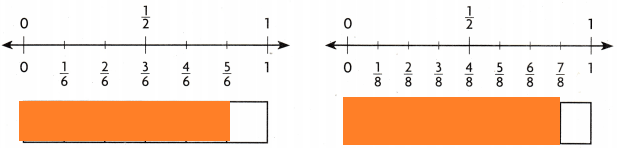Explanation:
$$\frac{5}{6}$$ = $$\frac{7}{8}$$ is the statement true
because their shaded parts are equal

Question 16.
Maggie did $$\frac{5}{12}$$ of her homework before dinner. Her brother did $$\frac{4}{10}$$ of his homework. Which statement is true about the fractions $$\frac{5}{12}$$ and $$\frac{4}{10}$$?(A) $$\frac{5}{12}$$ < $$\frac{4}{10}$$
(B) $$\frac{4}{10}$$ = $$\frac{1}{2}$$
(C) $$\frac{5}{12}$$ > $$\frac{4}{10}$$
(D) $$\frac{5}{12}$$ > $$\frac{1}{2}$$
Explanation:
$$\frac{5}{12}$$ > $$\frac{4}{10}$$ is the statement true

Question 17.
Multi-Step If you know that $$\frac{2}{6}$$ < $$\frac{1}{2}$$ and $$\frac{3}{4}$$ > $$\frac{1}{2}$$, what do you know
(A) $$\frac{3}{4}$$ = $$\frac{1}{2}$$
(B) $$\frac{2}{6}$$ > $$\frac{3}{4}$$
(C) $$\frac{2}{6}$$ > $$\frac{1}{2}$$
(D) $$\frac{2}{6}$$ < $$\frac{3}{4}$$
Explanation:
$$\frac{2}{6}$$ < $$\frac{3}{4}$$ is the statement
Both the fractions are compared with $$\frac{1}{2}$$

TEXAS Test Prep

Question 18.
Todd is using the benchmark $$\frac{1}{2}$$ to compare fractions. Which statement is NOT correct?
(A) $$\frac{5}{6}$$ < $$\frac{1}{2}$$
(B) $$\frac{3}{6}$$ = $$\frac{1}{2}$$
(C) $$\frac{5}{6}$$ > $$\frac{1}{2}$$
(D) $$\frac{5}{6}$$ ≠ $$\frac{1}{2}$$
Explanation:
$$\frac{5}{6}$$ < $$\frac{1}{2}$$ is the statement which is not true

### Texas Go Math Grade 4 Lesson 4.1 Homework and Practice Answer Key

Compare Fractions Using Benchmarks

Question 1.
How many sixths are equivalent to $$\frac{1}{2}$$?
Three sixths are equal to $$\frac{1}{2}$$
Explanation:
$$\frac{3}{6}$$ = $$\frac{1}{2}$$ we have to do the simplest form.

Question 2.
How can you compare $$\frac{7}{10}$$ and $$\frac{1}{2}$$ without using a model?
By doing the simplest form
five tenths are to $$\frac{1}{2}$$
Explanation:
so we can easily compare by simplest form.

Compare. Write < or >.

Question 3.
$$\frac{8}{10}$$ ___________ $$\frac{3}{8}$$
$$\frac{8}{10}$$ >  $$\frac{3}{8}$$
Explanation:
Both the fractions are compared with $$\frac{1}{2}$$

Question 4.
latex]\frac{1}{3}[/latex] _____________ $$\frac{7}{12}$$
latex]\frac{1}{3}[/latex] < $$\frac{7}{12}$$
Explanation:
Both the fractions are compared with $$\frac{1}{2}$$

Question 5.
$$\frac{2}{6}$$ _____________ $$\frac{7}{8}$$
$$\frac{2}{6}$$ <  $$\frac{7}{8}$$
Explanation:
Both the fractions are compared with $$\frac{1}{2}$$

Question 6.
$$\frac{3}{4}$$ _____________ $$\frac{1}{2}$$
$$\frac{3}{4}$$ > $$\frac{1}{2}$$
Explanation:
Both the fractions are compared with $$\frac{1}{2}$$

Question 7.
$$\frac{6}{6}$$ _____________ $$\frac{1}{3}$$
$$\frac{6}{6}$$ > $$\frac{1}{3}$$
Explanation:
Both the fractions are compared with $$\frac{1}{2}$$

Question 8.
$$}[/\frac{4}{5latex] _____________ [latex]\frac{1}{6}$$

$$}[/\frac{4}{5latex] > [latex]\frac{1}{6}[/latex Explanation: Both the fractions are compared with [latex]\frac{1}{2}$$

Find a numerator that makes the statement true.

Question 9.
$$\frac{2}{4}$$ > $$\frac{}{8}$$
$$\frac{2}{4}$$ > $$\frac{3}{8}$$
Explanation:
Both the fractions are compared with $$\frac{1}{2}$$

Question 10.
$$\frac{5}{10}$$ > $$\frac{}{8}$$
$$\frac{5}{10}$$ > $$\frac{3}{8}$$
Explanation:
Both the fractions are compared with $$\frac{1}{2}$$

Question 11.
$$\frac{3}{6}$$ > $$\frac{}{12}$$
$$\frac{3}{6}$$ > $$\frac{1}{12}$$
Explanation:
Both the fractions are compared with $$\frac{1}{2}$$

Question 12
$$\frac{2}{8}$$ > $$\frac{}{10}$$
$$\frac{2}{8}$$ > $$\frac{1}{10}$$
Explanation:
Both the fractions are compared with $$\frac{1}{2}$$

Problem Solving

Question 13.
Leticia read $$\frac{4}{5}$$ other book and Grace read $$\frac{6}{10}$$ of her book. Who read more of her book, Leticia or Grace? Explain.
Explanation:
Both the fractions are compared with $$\frac{1}{2}$$
$$\frac{6}{10}$$ is made as simplest form of $$\frac{3}{5}$$
$$\frac{3}{5}$$ and $$\frac{4}{5}$$ are compared so $$\frac{6}{10}$$  is lesser and $$\frac{4}{5}$$ is greater

Question 14.
Kyle made brownies and a cake. He cut the brownies into 6 equal parts and the cake into 8 equal parts. His family ate $$\frac{3}{4}$$ of the cake. If his family ate more cake than brownies, what fraction of the brownies could have been eaten?
$$\frac{3}{6}$$
Explanation:
$$\frac{3}{6}$$ is equal to $$\frac{1}{2}$$
and $$\frac{3}{4}$$ is greater than $$\frac{1}{2}$$

Lesson Check

Question 15.
Which symbol completes the following statement?
$$\frac{5}{8}$$ __________ $$\frac{9}{10}$$
(A) =
(B) ≠
(C) <
(D) >
Explanation:
$$\frac{5}{8}$$ < $$\frac{9}{10}$$
lesser than symbol is used

Question 16.
Garrett is using the benchmark $$\frac{1}{2}$$ to compare fractions. Which statement is NOT true?
(A) $$\frac{4}{8}$$ = $$\frac{1}{2}$$
(B) $$\frac{3}{8}$$ ≠ $$\frac{1}{2}$$
(C) $$\frac{3}{8}$$ < $$\frac{1}{2}$$
(D) $$\frac{3}{8}$$ > $$\frac{1}{2}$$
Explanation:
$$\frac{3}{8}$$ > $$\frac{1}{2}$$ is not correct statement.

Question 17.
Rob’s paper route is $$\frac{8}{10}$$ mile long. Lin’s route is $$\frac{3}{4}$$ mile long. What is true about $$\frac{8}{10}$$ and $$\frac{3}{4}$$?
(A) $$\frac{3}{4}$$ > $$\frac{8}{10}$$
(B) $$\frac{8}{10}$$ < $$\frac{3}{4}$$
(C) $$\frac{8}{10}$$ > $$\frac{3}{4}$$
(D) $$\frac{8}{10}$$ = $$\frac{3}{4}$$
Explanation:
$$\frac{8}{10}$$ > $$\frac{3}{4}$$ is the statement true about $$\frac{8}{10}$$ and $$\frac{3}{4}$$

Question 18.
Tia compares $$\frac{11}{12}$$ and $$\frac{2}{3}$$. Which statement
is true?
(A) $$\frac{2}{3}$$ = $$\frac{11}{12}$$
(B) $$\frac{2}{3}$$ > $$\frac{11}{12}$$
(C) $$\frac{11}{12}$$ = $$\frac{2}{3}$$
(D) $$\frac{2}{3}$$ < $$\frac{11}{12}$$
Explanation:
$$\frac{2}{3}$$ < $$\frac{11}{12}$$ is true about the statement of $$\frac{11}{12}$$ and $$\frac{2}{3}$$

Question 19.
Multi-Step Sandra is making crafts from leftover ribbons. She needs a ribbon longer than $$\frac{2}{3}$$ yard to make a bow. Which length of ribbon could she use for the bow?
(A) $$\frac{3}{4}$$ yard
(B) $$\frac{2}{6}$$ yard
(C) $$\frac{1}{5}$$ yard
(D) $$\frac{4}{7}$$ yard
Explanation:
$$\frac{3}{4}$$ yard of ribbon she use for the bow

Question 20.
Multi-Step Jessie has several bottles of used paint. He has three bottles of blue paint. The first bottle is $$\frac{1}{8}$$ full, the second bottle is $$\frac{1}{4}$$ full, and the third bottle $$\frac{1}{4}$$ is full He has one bottle of yellow paint that is $$\frac{1}{2}$$ full. Which of the following correctly compares the blue paint to the yellow paint?
(A) $$\frac{3}{8}$$ = $$\frac{1}{2}$$
(B) $$\frac{5}{8}$$ > $$\frac{1}{2}$$
(C) $$\frac{3}{8}$$ > $$\frac{1}{2}$$
(D) $$\frac{3}{8}$$ < $$\frac{1}{2}$$
$$\frac{5}{8}$$ > $$\frac{1}{2}$$
and compared with $$\frac{1}{2}$$